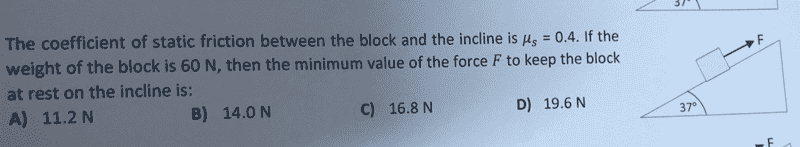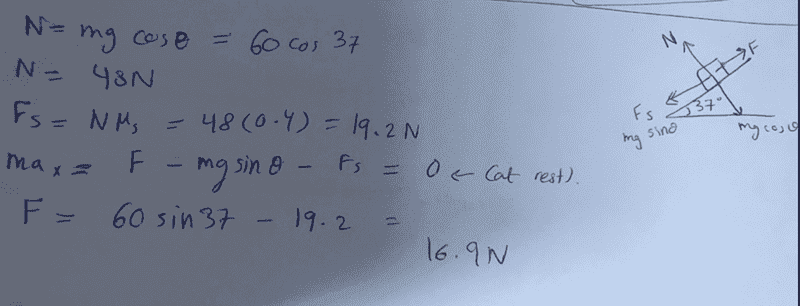# Newton's Law: block and incline

## Homework StatementFs= μs*N
ΣF=ma

## The Attempt at a Solution[/B]

## Answers and Replies

Orodruin
Staff Emeritus
Science Advisor
Homework Helper
Gold Member
What is your question?

Also, be advised that simply posting images of your work and of the problem is not compatible with the Homework guidelines of Physics Forums. Please type out the problem statement and your solution attempt in the future.

What is your question?

Also, be advised that simply posting images of your work and of the problem is not compatible with the Homework guidelines of Physics Forums. Please type out the problem statement and your solution attempt in the future.
thanks,
but question is that my answer is not an exact of choice C. so is my answer right?
sorry but my skills are not good to write math formula

TSny
Homework Helper
Gold Member
Hello.

I believe your final answer is correct. But it looks like you might have gotten lucky by having two compensating errors. Think about the direction of the friction force when the applied force is a minimum.

Orodruin
Staff Emeritus
Science Advisor
Homework Helper
Gold Member
but question is that my answer is not an exact of choice C. so is my answer right?
It is close enough to one of the answers to suspect that you (or the authors) have a rounding error somewhere.

sorry but my skills are not good to write math formula
Really the formulas here are so simple that you should have no problem typing them out. If you click the Σ symbol you also have a choice of different useful symbols for writing maths. You do not need to use LaTeX for formulas that are this simple.
Hello.

I believe your final answer is correct. But it looks like you might have gotten lucky by having two compensating errors. Think about the direction of the friction force when the applied force is a minimum.
By writing ##F = mg \sin(\theta) + F_s##, you are essentially writing "forces to the right = forces to the left". It is kind of artificially taking care of the signs by specifying all forces as positive and then placing them on the appropriate side of the equation. If I did not enter it wrong in Matlab, 16.9 N is the correct rounding of the answer, not 16.8 N.

TSny
Homework Helper
Gold Member
thanks,
but question is that my answer is not an exact of choice C. so is my answer right?
Some instructors like to use 37o in problems since the cosine and sine of this angle are very close to 4/5 = 0.80 and 3/5 = 0.60, respectively. (A "3 -4 -5" right triangle will have its smaller interior angle close to 37o.)

So, the author of this problem might have intended you to use 0.80 and 0.60 for the cosine and sine. See if that gives you an answer of 16.8.

Edit: When I was a student ages ago, we didn't have calculators. So it was more common in those days to use these approximations when working with a 37o angle.

•Orodruin
Orodruin
Staff Emeritus
Science Advisor
Homework Helper
Gold Member
Collecting all of your results symbolically, you would obtain
$$F = [60~\mbox{N}] \sin(\theta) - 0.4\cdot [60~\mbox{N}] \cos(\theta).$$
In comparison to the approximation mentioned by @TSny, ##\sin(37^\circ)## is slightly larger than ##3/5## and ##\cos(37^\circ)## is slightly smaller than 4/5. This indeed accounts for the difference.

Textbook authors should know better than to use more decimals than their precision allows.

TSny
Homework Helper
Gold Member
Textbook authors should know better than to use more decimals than their precision allows.
Yes.

Orodruin
Staff Emeritus
Science Advisor
Homework Helper
Gold Member
So let me correct this:
It is close enough to one of the answers to suspect that you (or the authors) have a rounding error somewhere.